## Covariant Tensor

A covariant tensor is a Tensor having specific transformation properties (c.f., a Contravariant Tensor). To examine the transformation properties of a covariant tensor, first consider the Gradient(1)

for which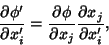(2)

where. Now let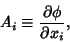(3)

then any set of quantities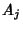which transform according to(4)

or, defining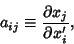(5)

according to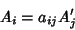(6)

is a covariant tensor. Covariant tensors are indicated with lowered indices, i.e.,.

Contravariant Tensors are a type of Tensor with differing transformation properties, denoted. However, in 3-D Cartesian Coordinates,(7)

for, 2, 3, meaning that contravariant and covariant tensors are equivalent. The two types of tensors do differ in higher dimensions, however. Covariant Four-Vectors satisfy(8)

whereis a Lorentz Tensor.

To turn a Contravariant Tensor into a covariant tensor, use the Metric Tensor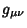to write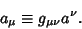(9)

Covariant and contravariant indices can be used simultaneously in a Mixed Tensor.

See also Contravariant Tensor, Four-Vector, Lorentz Tensor, Metric Tensor, Mixed Tensor, Tensor

References

Arfken, G. Noncartesian Tensors, Covariant Differentiation.'' §3.8 in Mathematical Methods for Physicists, 3rd ed. Orlando, FL: Academic Press, pp. 158-164, 1985.

Morse, P. M. and Feshbach, H. Methods of Theoretical Physics, Part I. New York: McGraw-Hill, pp. 44-46, 1953.